# Kinematics: Help and Review Chapter Exam

Exam Instructions:

Choose your answers to the questions and click 'Next' to see the next set of questions. You can skip questions if you would like and come back to them later with the yellow "Go To First Skipped Question" button. When you have completed the practice exam, a green submit button will appear. Click it to see your results. Good luck!

### Page 2

#### Question 9 9. Given this Velocity vs. Time graph, which of the following Position vs. Time graphs represents the same type of motion?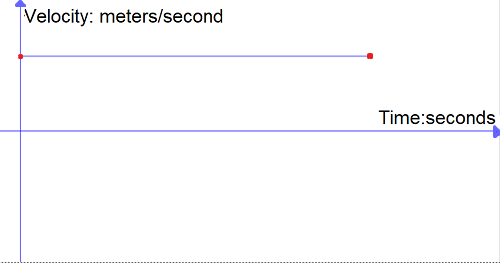#### Question 10 10. This graph represents an object that is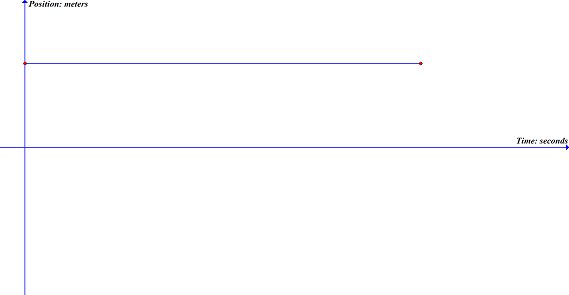### Page 3

#### Question 13 13. For this graph, calculate the average speed between 12 seconds and 20 seconds.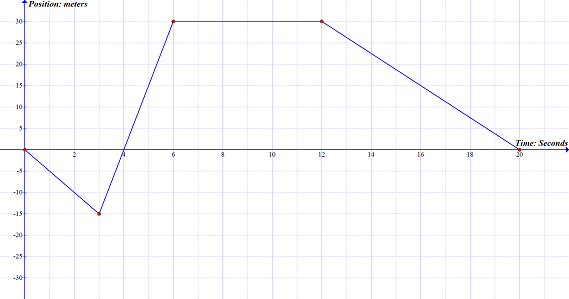#### Question 15 15. On this graph, what is the acceleration between 5 seconds and 8 seconds?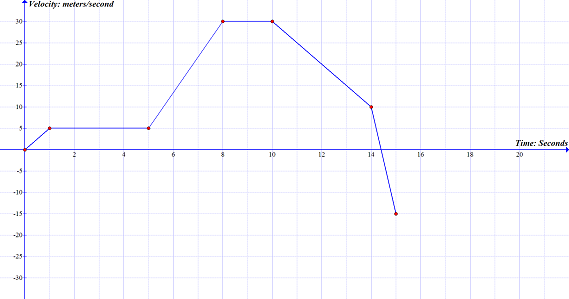### Page 4

#### Question 16 16. This graph is broken up into 5 sections, labeled A-E. On this graph, what section(s) represents positive acceleration?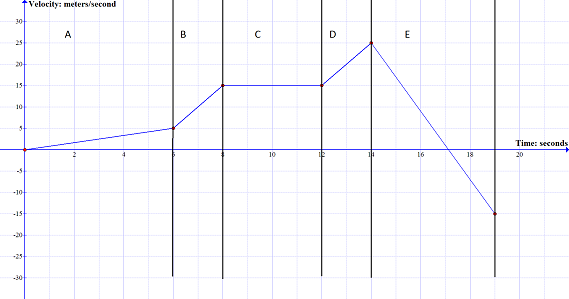#### Question 17 17. Using the graph, calculate the displacement for the section labeled A.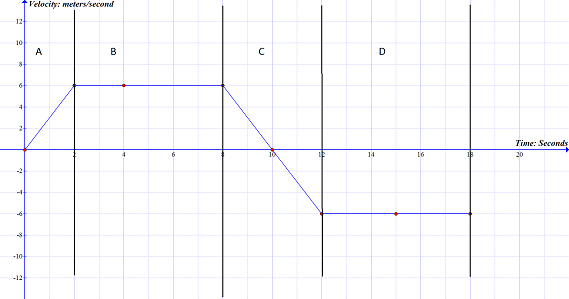### Page 5

#### Question 21 21. Given this Position vs. Time graph, which of the following Velocity vs. Time graphs represents the same type of motion?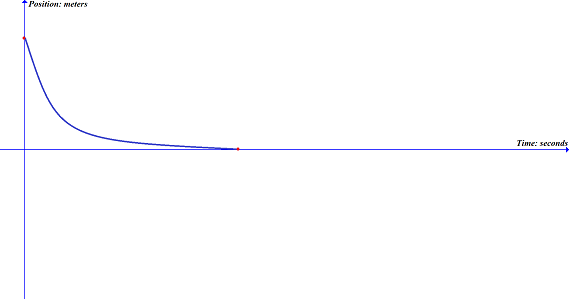#### Question 22 22. Using the graph, calculate the displacement for the section labeled B.#### Question 23 23. Calculate the slope of the line on the graph.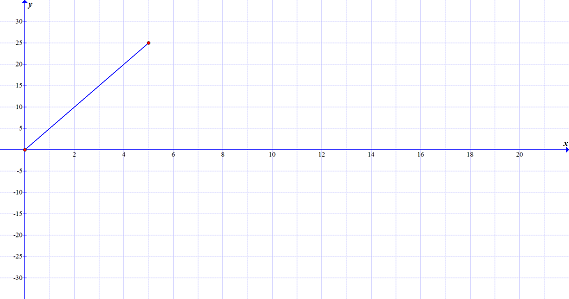#### Question 24 24. This graph represents how fast you jogged over a specific period of time. If you traveled 3 meters in 1 second, using the graph, what label should be at position B?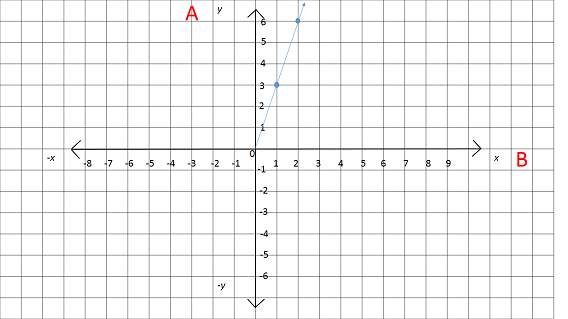### Page 6

#### Question 26 26. Looking at this graph, what assumption can you make about the displacement of the object over the time period indicated by the letter A?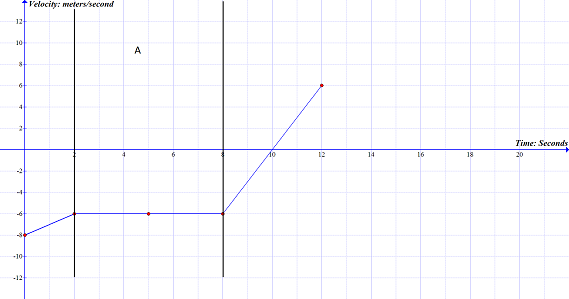#### Question 27 27. Using this graph, what is the distance of the object from the start point at 15 seconds?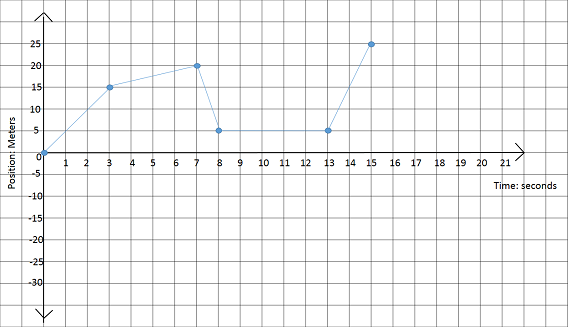#### Kinematics: Help and Review Chapter Exam Instructions

Choose your answers to the questions and click 'Next' to see the next set of questions. You can skip questions if you would like and come back to them later with the yellow "Go To First Skipped Question" button. When you have completed the practice exam, a green submit button will appear. Click it to see your results. Good luck!

Support